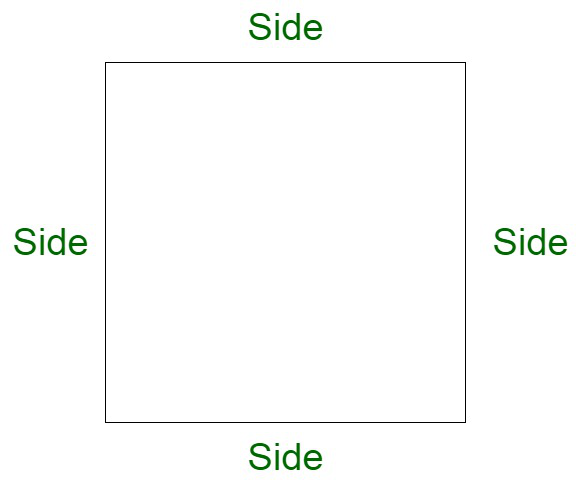# How to calculate the Perimeter of a Square?

• Last Updated : 07 Apr, 2022

Geometry is the branch of mathematics that deals with 2D and 3D figures, their shapes, sides, diagonals, area, volume, perimeter, and angles. The 2D figures include squares, rectangles, triangles, and circles. The 3D figures are also known as solids and it includes the cube, cuboid, hemisphere, etc.

• Vertices: They are the endpoints of a shape.
• Edge/Side: A line segment joining two adjacent vertices.
• Diagonals: A line segment joining two nonadjacent vertices.
• Area: Area is the space occupied by a figure.
• Volume: Volume is the capacity of a 3D figure.
• Angles: The point where two sides meet is the angle often measured in degrees or radians.

2D shapes are those which can be drawn on flat paper, hence it is often known as flat figures. They have sides and corners and some may also have curved boundaries. The parameters of 2D shapes are area and perimeter. Except for circles, the other shapes are known as polygons since A circle doesn’t have a side. A polygon that has all sides and angles equal is called the regular polygon.

### What is a Square?

A square is a closed regular polygon. In square all sides are equal and all angles are 90 degrees each. It is a 2 dimensional figure. It is a special type of quadrilateral.

A quadrilateral is a four-sided figure in which the sum of four internal angles is 360 degrees. In a square, the sum of 4 angles is 90×4 = 360 degrees. In the image of square ABCD where AC is the diagonal and AB is the side.A Square

Properties of a Square:

• A square has 4 sides equal
• Each angle in the square is 90 degrees.
• The diagonal of the square is √2 times the side.
• It is a two-dimensional figure
• The perimeter of a square is 4×side
• Area of the square is (side) 2
• The diagonal divides the square into two right-angled isosceles triangles.
• A square is a special case of a rectangle in which adjacent sides are equal.

### How to calculate the Perimeter of a Square?

Perimeter is the length of the boundary. It is often known as the distance around a closed 2D figure. The perimeters can be different according to the shapes given.

As we all know, perimeter is the total distance around a closed figure. Therefore perimeter of square is sum of lengths of 4 sides.

Perimeter = Side + Side + Side + Side = 4×side

Example:

Let us find the perimeter if side given is 12 m.

Perimeter = 4×12 = 48 m.

Finding the Perimeter of a Square if the side is not given.

• Using Diagonal

However if the side of square is not given but diagonal is given then the formula becomes,

Diagonal of a square,

d = √2×side

Therefore, the Perimeter of square is,

P = 4×(Diagonal/√2)

Example: Find the perimeter of the diagonal is 4√2 m.

Solution:

The Perimeter of square is,

P = 4×(Diagonal/√2)

= 4×(4√2/√2)

= 16 m

• Using Area

When the area of square is given. Let us assume the area of square is Area.

As we all know,

Area = (side)²

Therefore, the perimeter of the square is,

Perimeter = 4×√Area

Example: Find the perimeter if the area of the square is 49 square units.

Solution:

The Perimeter of square is,

P = 4×√Area

= 4×√49

= 28 units

### Sample Problems

Problem 1: Find the perimeter of the square if the side given is 4 units.

Solution:

The Perimeter of square is,

P = 4 × side

= 4 × 4

= 16 units

Problem 2: Find the side and perimeter of the square if the diagonal given is 2√2 cm.

Solution:

Side = Diagonal/√2

= 2√2/√2

= 2 cm

Perimeter of square = 4×side

= 8 cm

Problem 3: Find the side if the perimeter of the given square is √2.

Solution:

Perimeter = side × 4

√2 = side × 4

Therefore,

Side = √2/4

Problem 4: Find the diagonal if the perimeter of the square is 3√2 cm.

Solution:

Perimeter = 4×(diagonal/√2)

(3√2 × √2 ) /4 = diagonal

Diagonal = 1.5 cm

Problem 5: Find the perimeter of the square if its side is 1.5 cm.

Solution:

Perimeter = 4×side

= 4 × 1.5

= 6 cm

Problem 6: Find the perimeter if the area given is 25 sq units.

Solution:

Perimeter = 4√Area

= 4√25

= 20 units

Problem 7: Find the area and perimeter of the square if its side is 3 units.

Solution:

Perimeter = 4×side

= 4×3

= 12 units

Area = (Side)²

= (3)²

= 9 sq units

My Personal Notes arrow_drop_up